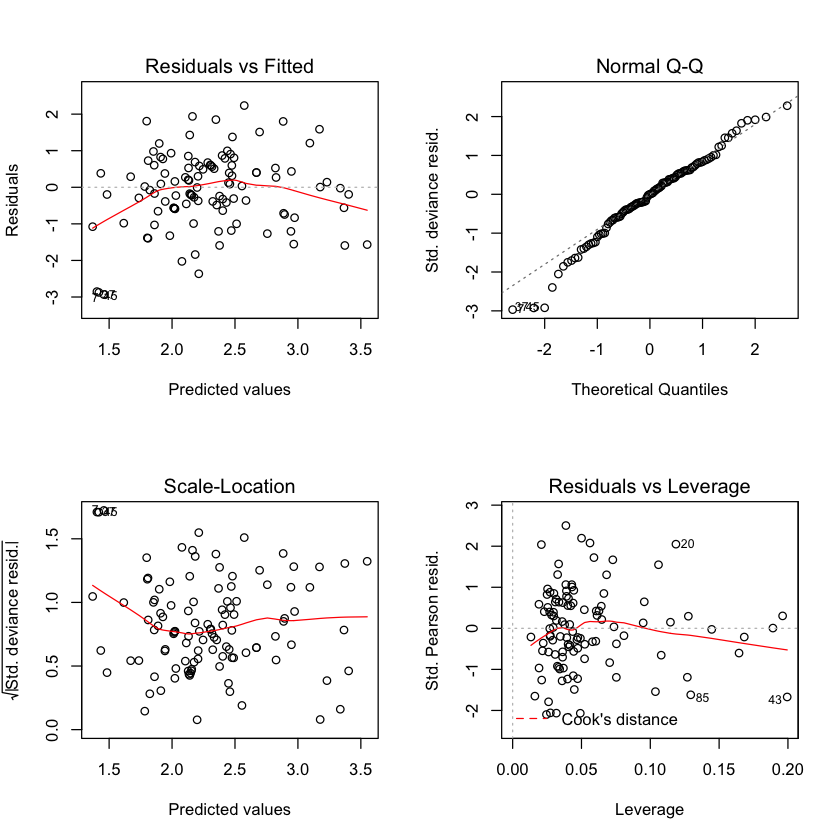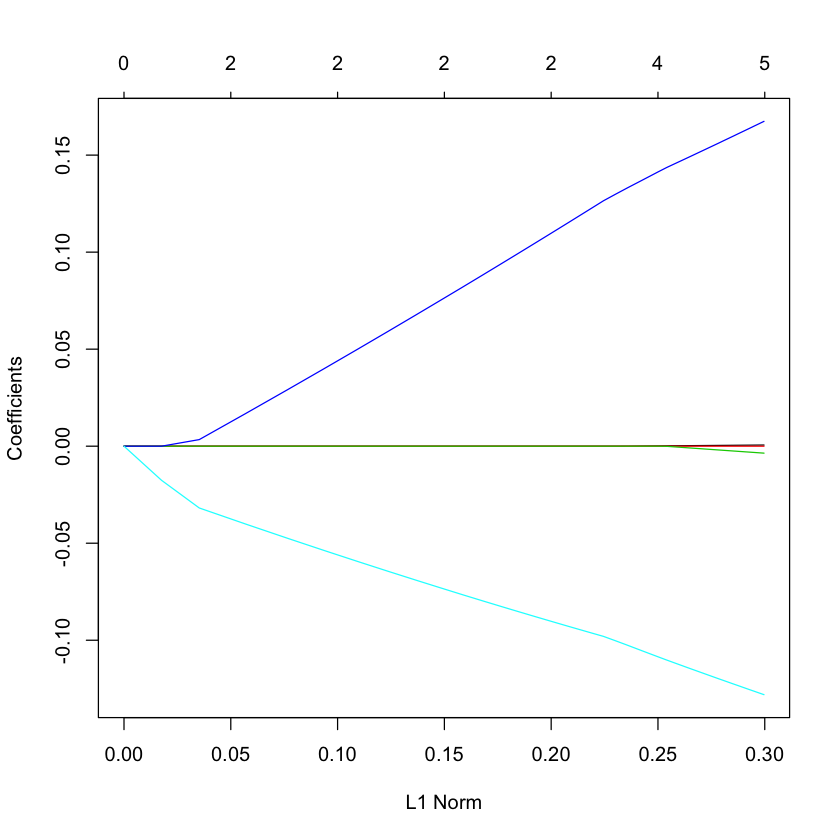# Poisson regression¶

• Contingency tables.
• Log-linear regression.
• Log-linear regression as a generalized linear model.

# Count data¶

## Afterlife¶

Men and women were asked whether they believed in the after life (1991 General Social Survey).

* Y N or U Total
M 435 147 582
F 375 134 509
Total 810 281 1091

Question: is belief in the afterlife independent of sex?

## Poisson counts¶

### Definition¶

• A random variable $Y$ is a Poisson random variable with parameter $\lambda$ if $$P(Y=j) = e^{-\lambda} \frac{\lambda^j}{j!}, \qquad \forall j \geq 0.$$
• Some simple calculations show that $E(Y)=\text{Var}(Y)=\lambda.$
• Poisson models for counts are analogous to Gaussian for continuous outcomes -- they appear in many common models.

## Contingency table¶

• Model: $Y_{ij} \sim Poisson(\lambda_{ij} )$.
• Null (independence): $H_0 :\lambda_{ij} = \delta \cdot \alpha_i \cdot \beta_j , \sum_i \alpha_i = 1, \sum_j \beta_j = 1.$
• Alternative: $H_a : \lambda_{ij} \in \mathbb{R}^+$
• Test statistic: Pearson’s $X^2$ : $X^2 = \sum_{ij} \frac{(Y_{ij}-E_{ij})^2}{E_{ij}} \overset{H_0}{\approx} \chi^2_1$
• Here $E_{ij}$ is the estimated expected value under independence.
• Why 1 df ? Independence model has 5 parameters, two constraints = 3 df. Unrestricted has 4 parameters.
• This is actually a regression model for the count data.
In :
Y = c(435,147,375,134)
S = factor(c('M','M','F','F'))
B = factor(c('Y','N','Y','N'))

N = sum(Y)
piS = c((435+147)/N,(375+134)/N)
piB = c((435+375)/N,(147+134)/N)

E = N*c(piS*piB, piS*piB, piS*piB, piS*piB)
# Pearson's X^2
X2 = sum((Y - E)^2/E)
c(X2, 1-pchisq(X2,1))

1. 0.162083972984318
2. 0.687245056382914

The independence test is called chisq.test in R. Depending on whether one corrects or not, we get the $X^2$ or a corrected version.

In :
chisq.test(matrix(Y,2,2), correct=FALSE)

	Pearson's Chi-squared test

data:  matrix(Y, 2, 2)
X-squared = 0.16208, df = 1, p-value = 0.6872

In :
chisq.test(matrix(Y,2,2))

	Pearson's Chi-squared test with Yates' continuity correction

data:  matrix(Y, 2, 2)
X-squared = 0.11103, df = 1, p-value = 0.739


## Contingency table as regression model¶

• Under independence \begin{aligned}  \log(E (Y_{ij} )) &= \log \lambda_{ij} = \log \delta + \log \alpha_i + \log \beta_j  \end{aligned}
• OR, the model has a log link.
• What about the variance? Because of Poisson assumption $Var(Y_{ij} ) = E (Y_{ij})$
• OR, the variance function is $V (\mu) = \mu.$

The goodness of fit test can also be found using a glm.

In :
summary(glm(Y ~ S + B, family=poisson()))

Call:
glm(formula = Y ~ S + B, family = poisson())

Deviance Residuals:
1        2        3        4
0.1394  -0.2377  -0.1494   0.2524

Coefficients:
Estimate Std. Error z value Pr(>|z|)
(Intercept)  4.87595    0.06787  71.839   <2e-16 ***
SM           0.13402    0.06069   2.208   0.0272 *
BY           1.05868    0.06923  15.291   <2e-16 ***
---
Signif. codes:  0 ‘***’ 0.001 ‘**’ 0.01 ‘*’ 0.05 ‘.’ 0.1 ‘ ’ 1

(Dispersion parameter for poisson family taken to be 1)

Null deviance: 272.685  on 3  degrees of freedom
Residual deviance:   0.162  on 1  degrees of freedom
AIC: 35.407

Number of Fisher Scoring iterations: 3


This model has the same fitted values as we had computed by hand above.

In :
fitted(glm(Y ~ S+B, family=poisson()))
E

1
432.098991750688
2
149.901008249313
3
377.901008249312
4
131.098991750687
1. 432.098991750688
2. 149.901008249313
3. 377.901008249313
4. 131.098991750687
• Here is the deviance test statistic.

• It is numerically close, but not identical to Pearson's $X^2$ for this data.

In :
DEV = sum(2*(Y*log(Y/E)+Y-E))
c(X2, DEV)

1. 0.162083972984318
2. 0.161995119420396

## Contingency table $(k \times m)$¶

• Suppose we had $k$ categories on one axis, $m$ on the other (i.e. previous example $k = m = 2$). We call this as $k \times m$ contingency table.
• Independence model $(H_0)$: $\log(E (Y_{ij} )) = \log \lambda_{ij} = \log \delta + \log \alpha_i + \log \beta_j$
• Test for independence: Pearson’s $$X^2 = \sum_{ij} \frac{(Y_{ij}-E_{ij})^2}{E_{ij}} \overset{H_0}{\approx} \chi^2_{(k-1)(m-1)}$$
• Alternative test statistic $G = 2\sum_{ij} Y_{ij} \log \left(\frac{Y_{ij}}{E_{ij}}\right)$

## Independence tests¶

• Unlike in other cases, in this case the full model has as many parameters as observations (i.e. it’s saturated).
• This test is known as a goodness of fit test.
• It tests: "how well does the independence model fit this data"?

• Unlike other tests we've seen, the deviance is the test statistic, not a difference of deviance.

## Lumber company example¶

• $Y$ : number of customers visting store from region;
• $X_1$ : number of housing units in region;
• $X_2$ : average household income;
• $X_3$ : average housing unit age in region;
• $X_4$ : distance to nearest competitor;
• $X_5$ : distance to store in miles.

## Poisson (log-linear) regression model¶

• Given observations and covariates $Y_i , X_{ij} , 1 \leq i \leq n, 1 \leq j \leq p$.
• Model: $$Y_{i} \sim Poisson \left(\exp\left(\beta_0 + \sum_{j=1}^p \beta_j X_{ij} \right)\right)$$
• Poisson assumption implies the variance function is $V (\mu) = \mu.$
In :
url = 'http://stats191.stanford.edu/data/lumber.table'
lumber.glm = glm(Customers ~ Housing + Income + Age + Competitor + Store,
family=poisson(), data=lumber.table)
summary(lumber.glm)

Call:
glm(formula = Customers ~ Housing + Income + Age + Competitor +
Store, family = poisson(), data = lumber.table)

Deviance Residuals:
Min        1Q    Median        3Q       Max
-2.93195  -0.58868  -0.00009   0.59269   2.23441

Coefficients:
Estimate Std. Error z value Pr(>|z|)
(Intercept)  2.942e+00  2.072e-01  14.198  < 2e-16 ***
Housing      6.058e-04  1.421e-04   4.262 2.02e-05 ***
Income      -1.169e-05  2.112e-06  -5.534 3.13e-08 ***
Age         -3.726e-03  1.782e-03  -2.091   0.0365 *
Competitor   1.684e-01  2.577e-02   6.534 6.39e-11 ***
Store       -1.288e-01  1.620e-02  -7.948 1.89e-15 ***
---
Signif. codes:  0 ‘***’ 0.001 ‘**’ 0.01 ‘*’ 0.05 ‘.’ 0.1 ‘ ’ 1

(Dispersion parameter for poisson family taken to be 1)

Null deviance: 422.22  on 109  degrees of freedom
Residual deviance: 114.99  on 104  degrees of freedom
AIC: 571.02

Number of Fisher Scoring iterations: 4

In :
par(mfrow=c(2,2))
plot(lumber.glm)## Interpretation of coefficients¶

• The log-linear model means covariates have multiplicative effect.
• Log-linear model model: $\frac{E(Y|\dots, X_j=x_j+h, \dots)}{E(Y|\dots, X_j=x_j, \dots)} = e^{h \cdot \beta_j}$
• So, one unit increase in variable $j$ results in $e^{\beta_j}$ (multiplicative) increase the expected count, all other parameters being equal.

## Generalized linear models¶

• Logistic model: ${\text{logit}}(\pi(X)) = \beta_0 + \sum_j \beta_j X_j \qquad V(\pi)=\pi(1-\pi)$
• Poisson log-linear model: $\log(\mu(X)) = \beta_0 + \sum_j \beta_j X_j, \qquad V(\mu) = \mu$
• These are the ingredients to a GLM …

## Deviance tests¶

• To test $H_0:{\cal M}={\cal M}_R$ vs. $H_a: {\cal M}={\cal M}_F$, we use $$DEV({\cal M}_R) - DEV({\cal M}_F) \sim \chi^2_{df_R-df_F}$$
• In contingency example ${\cal M}_R$ is the independence model $$\log(E(Y_{ij})) = \log\delta + \log \alpha_i + \log \beta_j$$ with ${\cal M}_F$ being the saturated model: no constraints on $E(Y_{ij})$.
In :
lumber.R.glm = glm(Customers ~ Housing + Income + Age,
family=poisson, data=lumber.table)
anova(lumber.R.glm, lumber.glm)
pchisq(263.45, 2, lower=FALSE, log=TRUE)

Resid. DfResid. DevDfDeviance
106 378.4343NA NA
104 114.9854 2 263.4488
-131.725
In :
1 - pchisq(263.45, 2)

0

## Model selection¶

• As it is a likelihood model, step can also be used for model selection.
In :
step(lumber.glm)

Start:  AIC=571.02
Customers ~ Housing + Income + Age + Competitor + Store

Df Deviance    AIC
<none>            114.98 571.02
- Age         1   119.36 573.40
- Housing     1   133.19 587.23
- Income      1   146.78 600.82
- Competitor  1   156.65 610.68
- Store       1   182.49 636.52

Call:  glm(formula = Customers ~ Housing + Income + Age + Competitor +
Store, family = poisson(), data = lumber.table)

Coefficients:
(Intercept)      Housing       Income          Age   Competitor        Store
2.942e+00    6.058e-04   -1.169e-05   -3.726e-03    1.684e-01   -1.288e-01

Degrees of Freedom: 109 Total (i.e. Null);  104 Residual
Null Deviance:	    422.2
Residual Deviance: 115 	AIC: 571
In :
step(glm(Customers ~ 1, data=lumber.table, family=poisson()), scope=list(upper=lumber.glm), direction='forward')

Start:  AIC=868.26
Customers ~ 1

Df Deviance    AIC
+ Store       1   184.41 632.45
+ Competitor  1   201.90 649.93
+ Housing     1   379.56 827.60
+ Income      1   399.15 847.19
<none>            422.22 868.26
+ Age         1   422.20 870.24

Step:  AIC=632.45
Customers ~ Store

Df Deviance    AIC
+ Competitor  1   149.33 599.37
+ Income      1   177.45 627.49
+ Housing     1   181.29 631.33
<none>            184.41 632.45
+ Age         1   183.19 633.23

Step:  AIC=599.37
Customers ~ Store + Competitor

Df Deviance    AIC
+ Income   1   134.98 587.02
<none>         149.33 599.37
+ Age      1   148.00 600.04
+ Housing  1   148.37 600.41

Step:  AIC=587.02
Customers ~ Store + Competitor + Income

Df Deviance    AIC
+ Housing  1   119.36 573.40
<none>         134.98 587.02
+ Age      1   133.19 587.23

Step:  AIC=573.4
Customers ~ Store + Competitor + Income + Housing

Df Deviance    AIC
+ Age   1   114.98 571.02
<none>      119.36 573.40

Step:  AIC=571.02
Customers ~ Store + Competitor + Income + Housing + Age


Call:  glm(formula = Customers ~ Store + Competitor + Income + Housing +
Age, family = poisson(), data = lumber.table)

Coefficients:
(Intercept)        Store   Competitor       Income      Housing          Age
2.942e+00   -1.288e-01    1.684e-01   -1.169e-05    6.058e-04   -3.726e-03

Degrees of Freedom: 109 Total (i.e. Null);  104 Residual
Null Deviance:	    422.2
Residual Deviance: 115 	AIC: 571

# LASSO¶

• LASSO also applicable
In :
library(glmnet)
X = model.matrix(lumber.glm)[,-1]
Y = lumber.table\$Customers
G = glmnet(X, Y, family='poisson')
plot(G)

Loading required package: Matrix